Math Worksheets for Kids Home > Pre-made Math Worksheets - Fifth Grade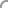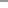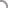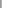Welcome Guest User
 • Spotlight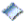New Worksheets...Easter Worksheets...Custom Search
 Pre-made Worksheets: By Grade  — Pre-K and Kindergarten  — 1st Grade  — 2nd Grade  — 3rd Grade  — 4th Grade  — 5th Grade   By ThemeValentine's DaySt. Patrick's DayEasterSpringSummerFallHalloweenThanksgivingChristmasNew Year   By OperationCounting/ Skip CountingComparisonAdditionSubtractionMultiplicationDivisionMatching   By Special Topics/ Skills  — Basic Numbers  — Number Lines  — Fact Family  — Place Value   — Time   — Thermometer   — Money  — Even/Odd Numbers   — Roman Numbers  — Prime Numbers  — Sort/Order Numbers  — Measurement  — Color By Number  — Word problems  — Ordinal Number & Names  — Rounding  — Percentage  — Fractions  — Decimals   — Algebra  — Shapes/Geometry  — Math Drills Custom Worksheets: • Standard Operators    Addition/Subtraction    Multiplication/Division • Number Tables • Math Fact Table Interactive Flash Cards • Multiplication • Addition Other Activities • Quiz/Assessments Contact Us • Suggestions Notify Me >> Enter Email: Testimonial ... Fabulous! My son didn't understand Fact Families at school - and after I found your site, he mastered the concept very quickly... Texas, USA Tell us what do you think about our site.>>
 Explore Schools in USA AboutUs.org Page MathCentral4Kids Site Federal Education Regulations California Law on Education Other sites: A B C D FR F G H IAirlines Scottsdale Deals Privacy Policy Terms of Use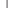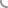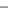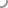Select a Worksheet

Categories:
 1. Addition 2. Subtraction 3. Fractions 4. Multiplication 5. Division 6. Mixed Operations 7. Rounding 8. Power 9. Geometry 10. Algebra 11. Summer Practice Note: - Click here for Spring Math Worksheets.- Click here to create Custom and Mixed Worksheets. - Click here for Math Drills Worksheets.

Single Digit - Set 3

Tenths (Level B)
Hundredths (Level B)
Thousandths (Level B)
Mixed - Tenths, Hundredths, Thousandths (Level B)

 2 Subtraction Top

Subtract a number

Subtraction - Decimal Numbers
Tenths (Level B)
Hundredths (Level B)
Thousandths (Level B)
Mixed (T, H, Th) (Level C)

 3 Fractions Top

Convert - Fractions to Decimals
Level A4 - Proper Fraction, Denominator 2, 4, 5, 10
Level A5 - Proper Fraction, Denominator 2, 4, 5, 10, 20, 25, 50, 100
Level A6 - Proper Fraction, Denominator 2 to 100 and 1000.
Level B1- Proper/Improper/Mixed Fractions, Denominator 10,100,1000
Level B2- Proper/Improper/Mixed Fractions, Denominator 2 to 100 and 1000.

Reduce Fractions to Lowest Terms
Level D1 (Simple)
Level D2 (Medium)
Level D3 (Hard)

Fraction Addition (Mixed - Common and Uncommon denominators)
Level D1 (Simple)
Level D2 (Medium)
Level D3 (Hard)
Level D4 (Harder)

Level D5 (Harder)

 4 Multiplication Top

Multiplication : Single Digit
Tables of 0 to 5 ([0 - 5] X [0 - 9])
Tables of 0 to 6 ([0 - 6] X [0 - 9])
Tables of 0 to 7 ([0 - 7] X [0 - 9])
Tables of 0 to 8 ([0 - 8] X [0 - 9])
Tables of 0 to 9 ([0 - 9] X [0 - 9])

Squares
(5 to 25)

Multiplication - Basic Drills
One Digit (0 to 9)
One Digit (0 to 12)
1-2 Digits (#'s from 6 to 12)
Tough Ones (Selective #'s from 4 to 12)

 5 Division Top

Division - Basic Drills
Single Digit Divisor, without Remainder

 6 Mixed Operations Top

Drills - Mixed Operators - Addition, Subtraction
Single Digit

Drills - Mixed Operators - Addition, Multiplication
Single Digit

Drills - Mixed Operators - Addition, Subtraction, Multiplication
Single Digit

Drills - Mixed Operators - Multiplication, Division
Single Digit

Drills - Mixed Operators - Addition, Subtraction, Multiplication, Division
Single Digit

 7 Rounding Top

Round to nearest hundredth

 8 Power Top

Power
Power of 10

 9 Geometry Top

Circle
Area
Circumference
Area & Circumference

 10 Algebra Top

Simplify Expression
Level 1
Level 2

Solve the Equation (1 variable)
Level 1 (e.g. x + 1 = 9)
Level 2 (e.g. 3x = 9)
Level 3 (e.g. x + 9 - 8 = 12)
Level 4 (e.g. 7x - 8 + 6 = 33)

 11 Summer Practice Top

Summer Practice Part 1 - Addition (0 to 9)

Summer Practice Part 2 - Subtraction (0 to 9)

Summer Practice Part 3 - Multiplication (0 to 9)

Summer Practice Part 4 - Division (0 to 9)

Summer Practice Part 5 - Addition (0 to 12)

Summer Practice Part 6 - Subtraction (0 to 12)

Summer Practice Part 7 - Multiplication (0 to 12)

Summer Practice Part 8 - Division (0 to 12)

 Want to get notified about new myWeblets worksheets?
Search for Worksheets: